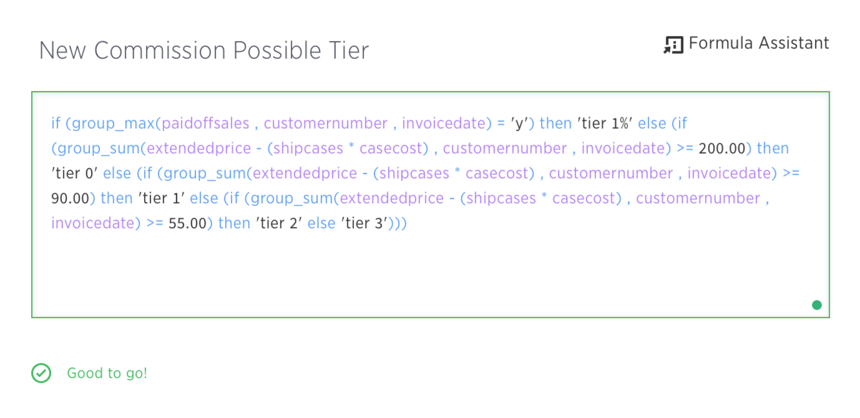# Compress Group_Sum

So I am attempting to create a formula for our salespeople that shows various "tiers" for commission - for example, if said salesperson made >= X dollars in Gross Profit on a customer for that day, they are paid a commission of 23.5%. If they make higher than Y dollars it bumps up to 25%, and so on.

What I have done is label these as 'tier 1', 'tier 2', etc. for easy readability. However, I run into a problem here. The way the commission is determined is based off of the total gross profit for a customer for a day, even if multiple invoices that day were sold with GP less than X dollars.

As a practical example, suppose the cutoff for 23.5% is \$30 GP. If Invoice 1 makes \$23 GP, it earns no commission on it (didn't meet the \$30 GP mark). However, if another invoice is issued to the same customer on the same day (let's call it Invoice 2) for \$10 GP, then the total GP for that customer for that day adds up to \$33 GP. Now it is above the cutoff threshold, and commission is paid at 23.5% for both invoices' GP.

So, in order to calculate this, what I have done is create the following formula:So essentially this groups together gross profits by customer number then by invoice date, allowing me to break apart data from a tier level to an customer level to an invoice level and get the same results.

Now, I want to graph these tiers as a percentage - essentially calculating the total number of invoices where the tier = 'tier 0' or tier = 'tier 1' and so forth. But when I do that, because of the group_sum there is no way to condense the numbers back down so that I end up with 5 tiers and the count of invoices for those tiers.

My question is: is there a way that I can find the number of invoices where the tier = tier 0, while making sure the invoices are properly labeled based off of gross profit for multiple invoices for the same customer on the same day?

Thanks in advance! Let me know if there is anything you don't understand about the question.

3replies Oldest first
• Oldest first
• PopularLike Follow